# Logarithms

In this Mentimeter template the concept of logarithms are elaborated on. The template is designed primarily as an introduction but can also be used in the middle or as a form of reinforcement at the end of a subject block surrounding exponential functions.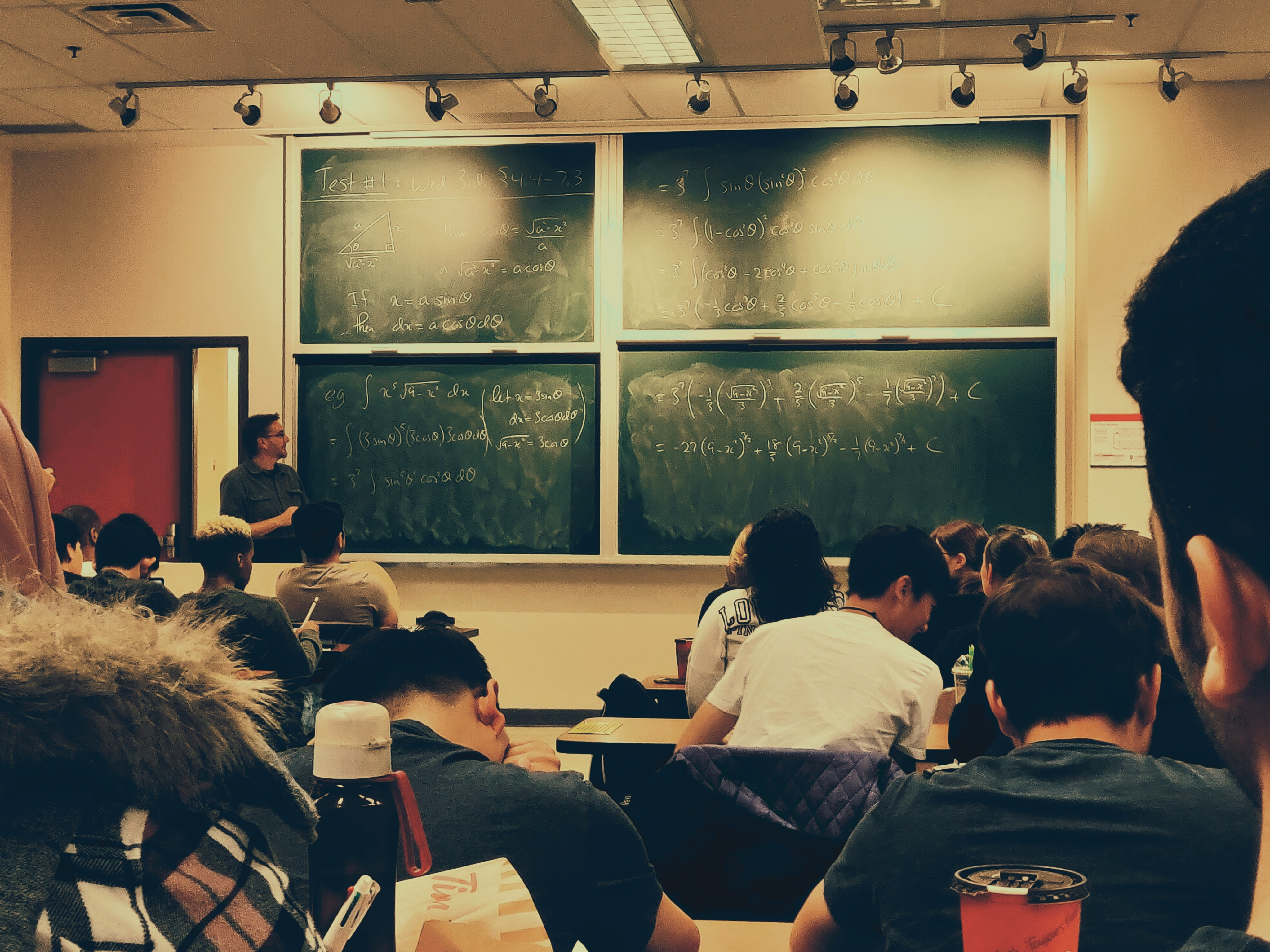## How to use the template

The students need a pencil and paper for some of the questions in the presentation. Here follows some ideas on how the slides are intended to be used but feel free to use the template however you like. Included in each cell is the purpose and other information that could be of use for you as a teacher. A general idea regarding all questions is that the students should work after the model Think-Pair-Share (TPS). When implementing TPS the students should first answer the question individually, then discuss in pairs (or groups of three) followed by a classroom discussion. As a concluding remark. The content in the slides are designed primarily for developing the students understanding of mathematical concepts rather than the procedural "know how". Therefore discussions and questions from the students is a central part of using the presentation where your expertise as a teacher is central to clarify misconceptions and lead the classroom discussions.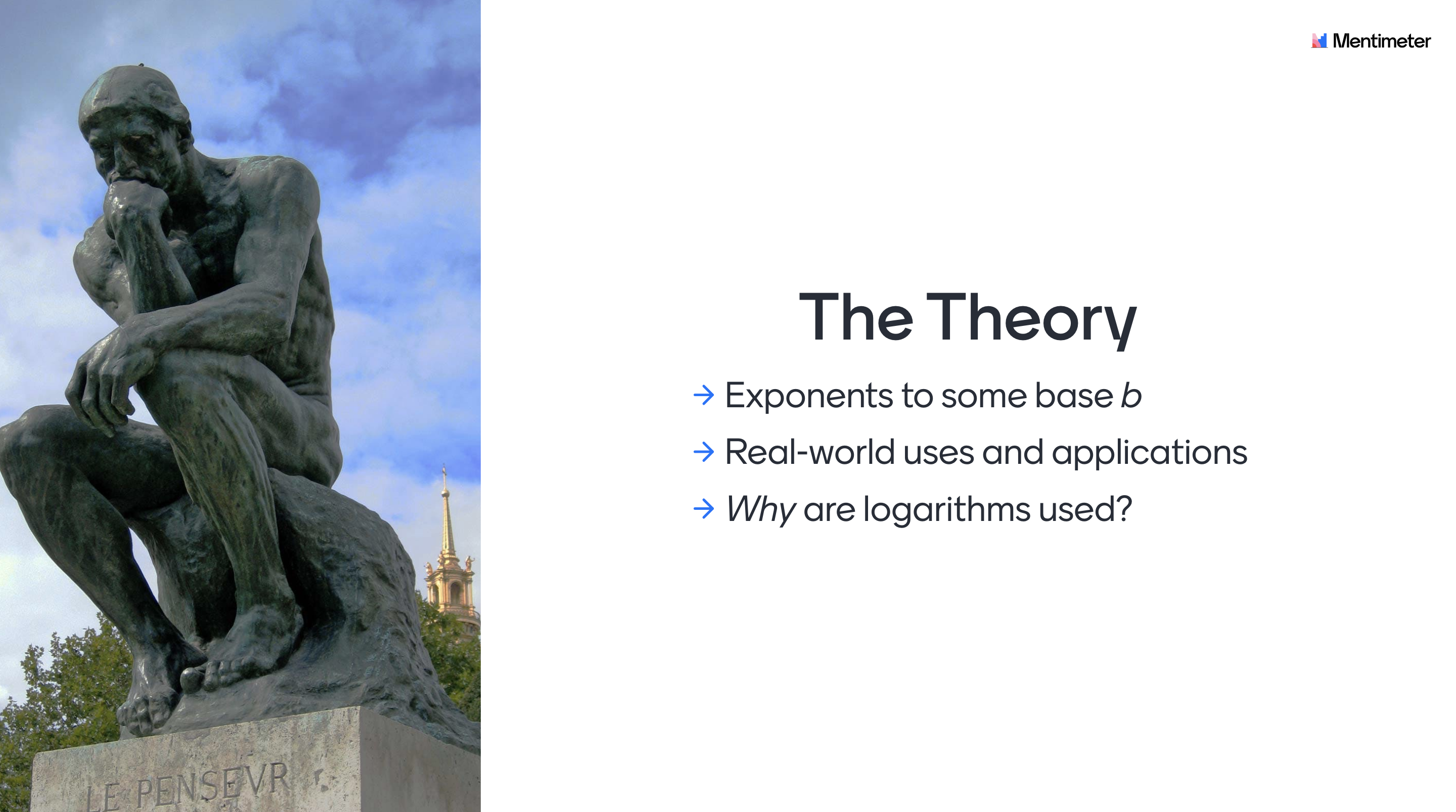## Theoretical background

Logarithms can be defined as exponents to some base b, but for a student such a definition could confuse and gives no insights on where and why the concepts of logarithms are being used and applied in a daily context. The purpose of this module is to give an understanding as to why logarithms are used.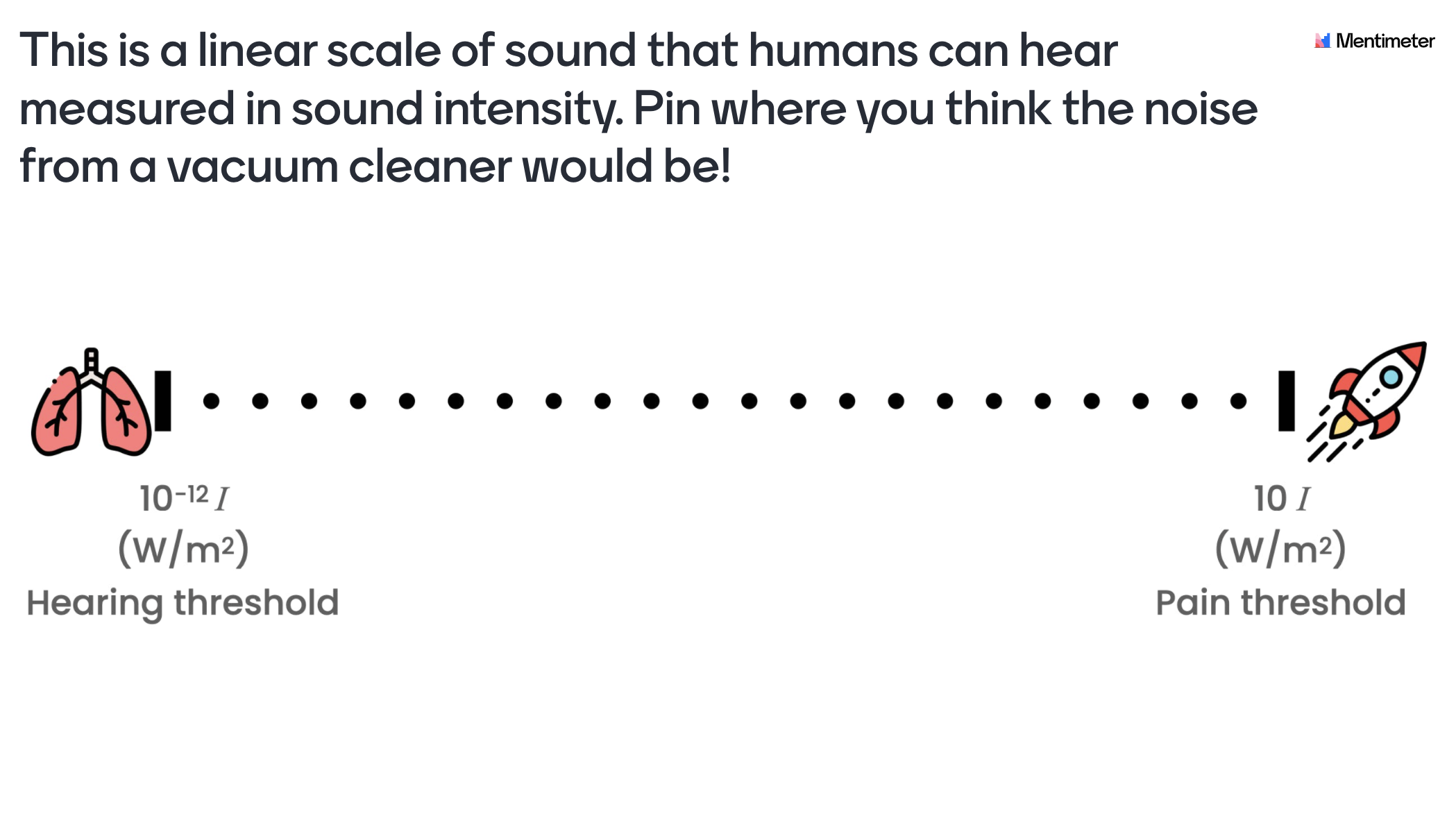Slide 1 - Introduction: Introduce the theme of the lecture. For example you could mention that the concept of logarithms could be applied in the real world as a "tool" for handling a large set of numerical data.

Slide 2 - General information: Inform the students regarding the TPS methodology and how they can access the presentation.

Slide 3 - Criteria for success: Introduce a "criteria for success" for the lesson with a problem that will be revisited and solved at the end of the lesson. The idea here is to make the learning visible, so the students can see for themselves that they’ve actually learned something.

Slide 4 - Sound is energy!: The purpose of this video is to demonstrate that sound can be thought of as energy.

Slide 5 - Where would you place the vacuum cleaner on a linear scale of sound intensity?: This will give the students a first visual representation with a linear scale of sound intensity and how they perceive the sound strength of a vacuum cleaner in relation to "threshold of hearing" and "pain threshold".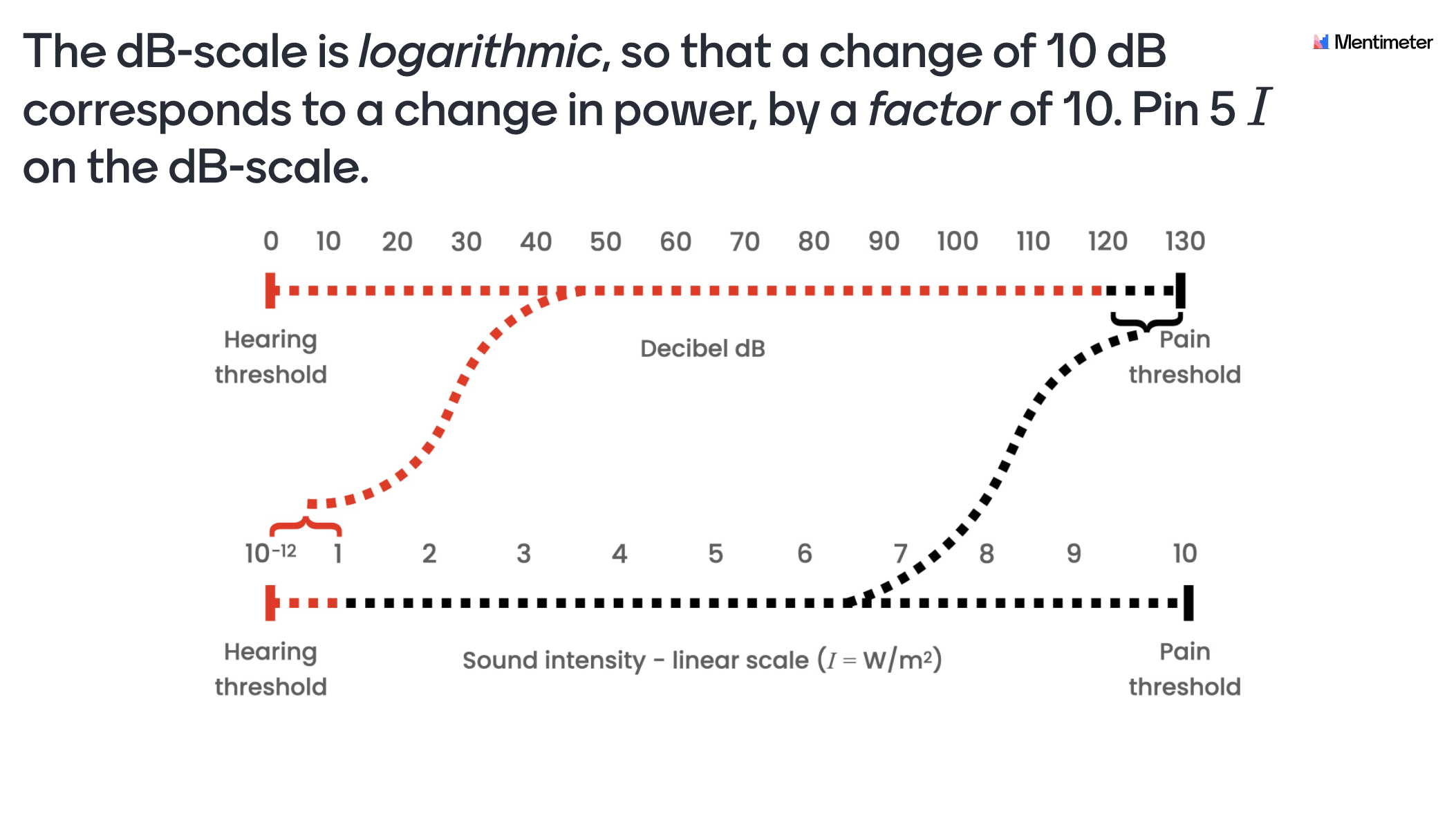Slide 6 - This scale makes no sense!: The idea here is to give the students a visual representation of where sounds that they encounter in their daily life could be placed on a linear scale of sound intensity. It introduces the problem of using a non-logarithmic scale when values differ by a large magnitude.

Slide 7 - How could we make this scale more sensible?: The purpose for this slide is to get the student to reason about the advantages of logarithms. A clue for the students would be to use the exponent.

Slide 8 - Three scales of sound intensity!: Introducing the Decibel-, linear- and the logarithmic scale simultaneously to visualize the difference of using a linear and a logarithmic scale.

Slide 9 - The Decibel scale is logarithmic!: The purpose of this slide is to see if the student has grasped the idea that you could "transfer" values from a linear scale to a logarithmic scale.

Slide 10 - A better scale - the Decibel scale!: This is a content slide where the students get the opportunity to see where the previously discussed sounds in the template are being located in a Decibel scale.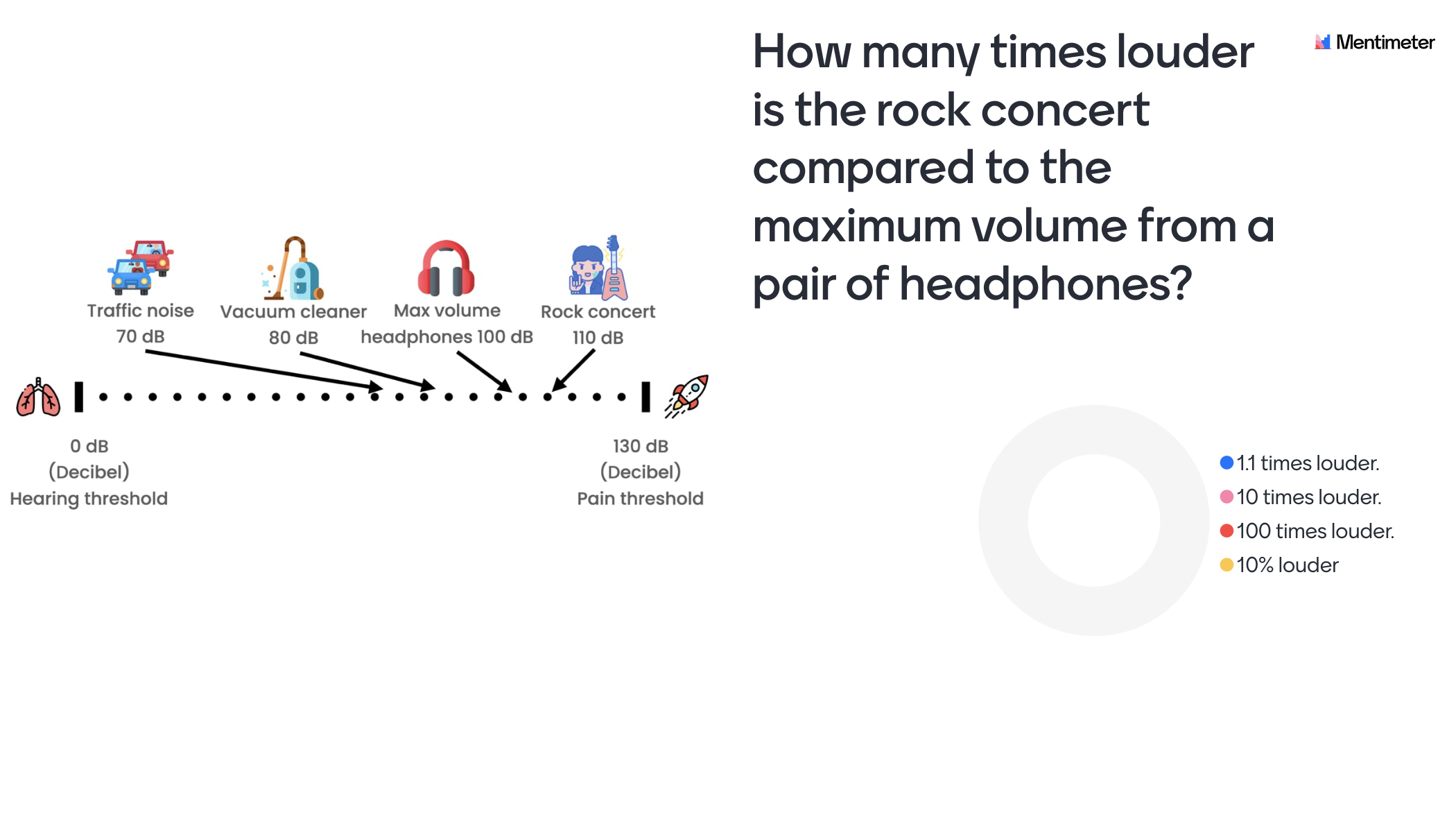Slide 11 - How many times louder is the rock concert compared to the headphones?: This slide will test if the students have grasped the content of the previous slides. It will give the teacher an idea if the students has grasped that a "linear" increase or decrease in the Decibel scale is actually not the same when looking at the corresponding linear scale.

Slide 12 - What is the ratio between the rock concert and a vacuum cleaner?: The students get another opportunity to strengthen what an increase in the Decibel scale translates to when looking at an increase of sound intensity. But this time the students need to recall the ratio concept.

Slide 13 - What is a log scale?: The purpose here is to gather the class to clarify that a "log scale" is a way of displaying numerical data in a more comprehensible way. To see that everyone is onboard.

Slide 14 - Repetition of base, exponent and exponentiation: Repetition for the students to prepare them for some calculation tasks in the coming slides and the quiz.Slide 15 - What is x in the example?: Here the students start applying logarithms with classical mathematical notation and get a chance to practice simple arithmetics.

Slide 16 - Criteria for success revisited: Return to the problem that was introduced in the beginning of the lecture to make the students realize they’ve actually learnt something.

Slide 17 - Q&A: Let the students individually write down what they still feel is unclear about logarithms.

Slide 18-24 - Quiz Competition: Ask the student to enter their real names. Let the students compete in a fun way while you as a teacher can get a sense of the individual level of knowledge. This information could be used to give support for students in need of guidance.